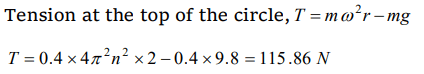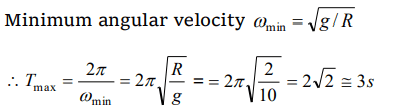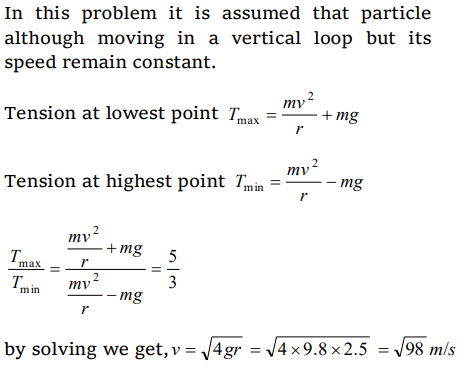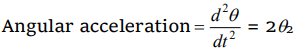## Motion in a Plane Questions and Answers Part-16

1. A particle is kept at rest at the top of a sphere of diameter 42 m. When disturbed slightly, it slides down. At what height ‘h’ from the bottom, the particle will leave the sphere
a) 14 m
b) 28 m
c) 35 m
d) 7 m

Explanation:2. The coordinates of a moving particle at any time ‘t’ are given by $x=\alpha t^{3}$ and $y=\beta t^{3}$. The speed of the particle at time ‘t’ is given by
a) $\sqrt{\alpha^{2}+\beta^{2}}$
b) $3 t\sqrt{\alpha^{2}+\beta^{2}}$
c) $3 t^{2}\sqrt{\alpha^{2}+\beta^{2}}$
d) $t^{2}\sqrt{\alpha^{2}+\beta^{2}}$

Explanation:3. A small disc is on the top of a hemisphere of radius R . What is the smallest horizontal velocity v that should be given to the disc for it to leave the hemisphere and not slide down it ? [There is no friction]
a) $v=\sqrt{2gR}$
b) $v=\sqrt{gR}$
c) $v=\frac{g}{R}$
d) $v=\sqrt{g^{2}R}$

Explanation: $v=\sqrt{gR}$

4. A body of mass 0.4 kg is whirled in a vertical circle making 2 rev/sec. If the radius of the circle is 2 m, then tension in the string when the body is at the top of the circle, is
a) 41.56 N
b) 89.86 N
c) 109.86 N
d) 115.86 N

Explanation:5. A bucket full of water is revolved in vertical circle of radius 2m. What should be the maximum timeperiod of revolution so that the water doesn't fall off the bucket
a) 1 sec
b) 2 sec
c) 3 sec
d) 4 sec

Explanation:6. The maximum and minimum tension in the string whirling in a circle of radius 2.5 m with constant velocity are in the ratio 5 : 3 then its velocity is
a) $\sqrt{98}$ m/s
b) $7 m\diagup s$
c) $\sqrt{490}$ m/s
d) $\sqrt{4.9 }$ m/s

Explanation:7. For a particle in circular motion the centripetal acceleration is
a) Less than its tangential acceleration
b) Equal to its tangential acceleration
c) More than its tangential acceleration
d) May be more or less than its tangential acceleration

Explanation: There is no relation between centripetal and tangential acceleration. Centripetal acceleration is must for circular motion but tangential acceleration may be zero.

8. A particle moves in a circular path with decreasing speed. Choose the correct statement
a) Angular momentum remains constant
b) Acceleration $\overrightarrow{a}$ is towards the center
c) Particle moves in a spiral path with decreasing radius
d) The direction of angular momentum remains constant

Explanation: Angular momentum is a axial vector. It is directed always in a fix direction (perpendicular to the plane of rotation either outward or inward), if the sense of rotation remain same

9. A body of mass 1 kg is moving in a vertical circular path of radius 1m. The difference between the kinetic energies at its highest and lowest position is
a) 20J
b) 10J
c) $4\sqrt{5 }$ J
d) $10\left(\sqrt{5-1}\right) J$

10. The angle turned by a body undergoing circular motion depends on time as $\theta=\theta_{0}+\theta_{1}t+\theta_{2}t^{2}$   . Then the angular acceleration of the body is
a) $\theta_{1}$
b) $\theta_{2}$
c) $2\theta_{1}$
d) $2\theta_{2}$